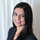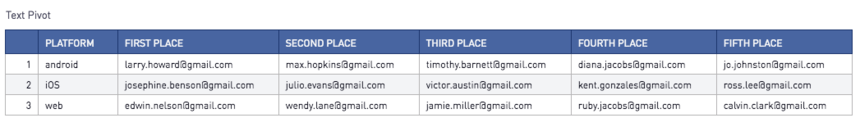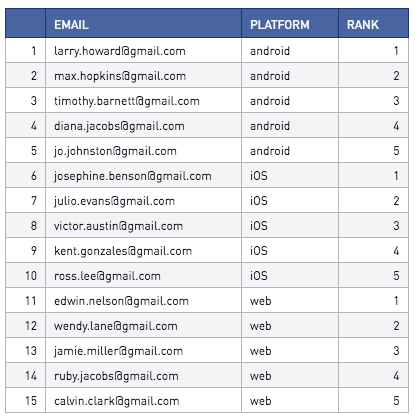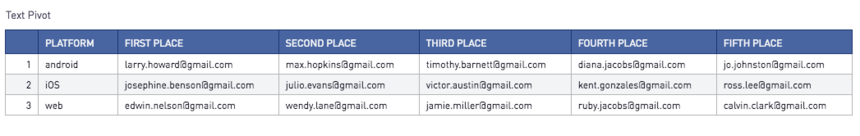# Text Pivots - Pandas****The code for this solution is leveraged from this source ***

Let's say you want to pivot a table, but you want the actual data in the pivoted cells to be a string.

Take this SQL output as an example. Note, if your data doesn't already have a rank built in and you want to calculate this instead, use a window function!). We want to transform this table so the ranks are displayed as columns, and the email addresses are displayed as values.Here is our desired output:We can accomplish this using the following Python 3.6 code

```# SQL output is imported as a pandas dataframe variable called "df"
import pandas as pd

#Pivot the table. Source: http://www.enricobergamini.it/python-pivot-table/
pivot_table = df.pivot_table(index=['platform'],
columns=['rank'],
values=['email'],
aggfunc=lambda x: ' '.join(str(v) for v in x))

#Reset the index and rename columns
pivot_table = pivot_table.reset_index()
pivot_table.columns = ['platform', 'first place', 'second place', 'third place', 'fourth place', 'fifth place']

# Use Periscope to visualize a dataframe or an image by passing data to periscope.output()
periscope.output(pivot_table)
```